1.800.221.5175
Core Program
Full Access
Core Program
Full Access
High-Impact Tutoring
Core Program
Full Access
National Program
Full Access
New York
Critical Thinking for Active Math Minds
Preparing for Standards Based Assessments
Vocabulary Workshop, Tools for Comprehension Grades 1–5
Print Program
Interactive Edition
Print Program
Interactive Edition
Vocabulary Workshop, Tools for Excellence Grades 6–12+
Print Program
Interactive Edition
Vocabulary for Success
Progress English Language Arts Grades K–8
National Program
Full Access
High-Impact Tutoring
New York
Grammar Workshop, Tools for Writing
Grammar for Writing
Print Program
Interactive Practice Bundle
Fluency Booster Practice Book
Interactive Assessments
High-Impact Tutoring
Building Reading Success with Wiley Blevins
Print Program
Interactive Edition
Professional Learning

## How Math Games and Activities Increase Student Proficiency in Grade 3

Math games and activities can be an excellent tool to supplement and support math instruction. Using math games in your classroom allows students to practice mathematics in fun but also impactful ways. Students love games because they are engaging and exciting, and teachers love games because they help students practice what they've learned.

Students in Grade 3 will learn about multiplication, division, and practice strategies for multiplication and division within 100. They will also develop an understanding of fractions and know how to analyze two-dimensional shapes using counters, base-ten blocks, coins, decimal squares, measuring tools, pattern blocks, area models, and number lines.

## Pattern Blocks

### Much research suggests that concrete activities facilitate students’ geometric thinking. This instruction model can be used to have students subdivide, combine, and transform shapes, investigate the relationships among shapes, and find perimeter and area.

Example A: Combine pattern blocks to make a shape. Then, recombine them to make another shape.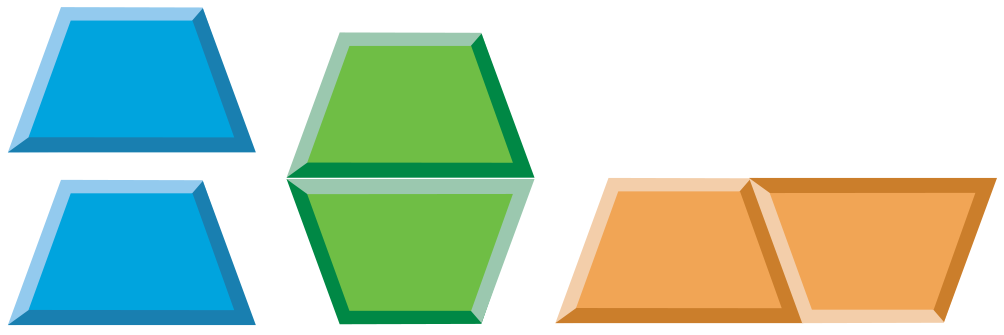For each new shape, have students complete a table to display its properties and write a description in words.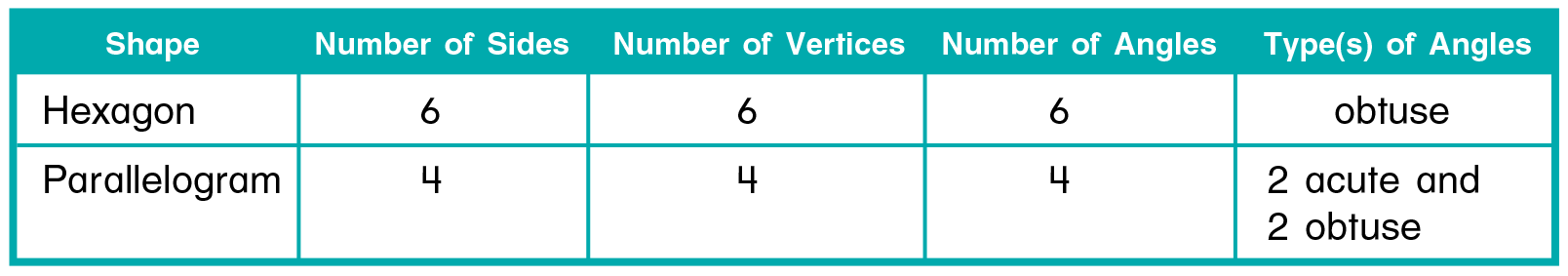A hexagon is a polygon with 6 sides.
A parallelogram is a quadrilateral with opposite sides that are parallel and equal.

Example B: Trace the new shapes on 1/4-inch grid paper and estimate their perimeter and area by counting squares along their sides and squares inside each.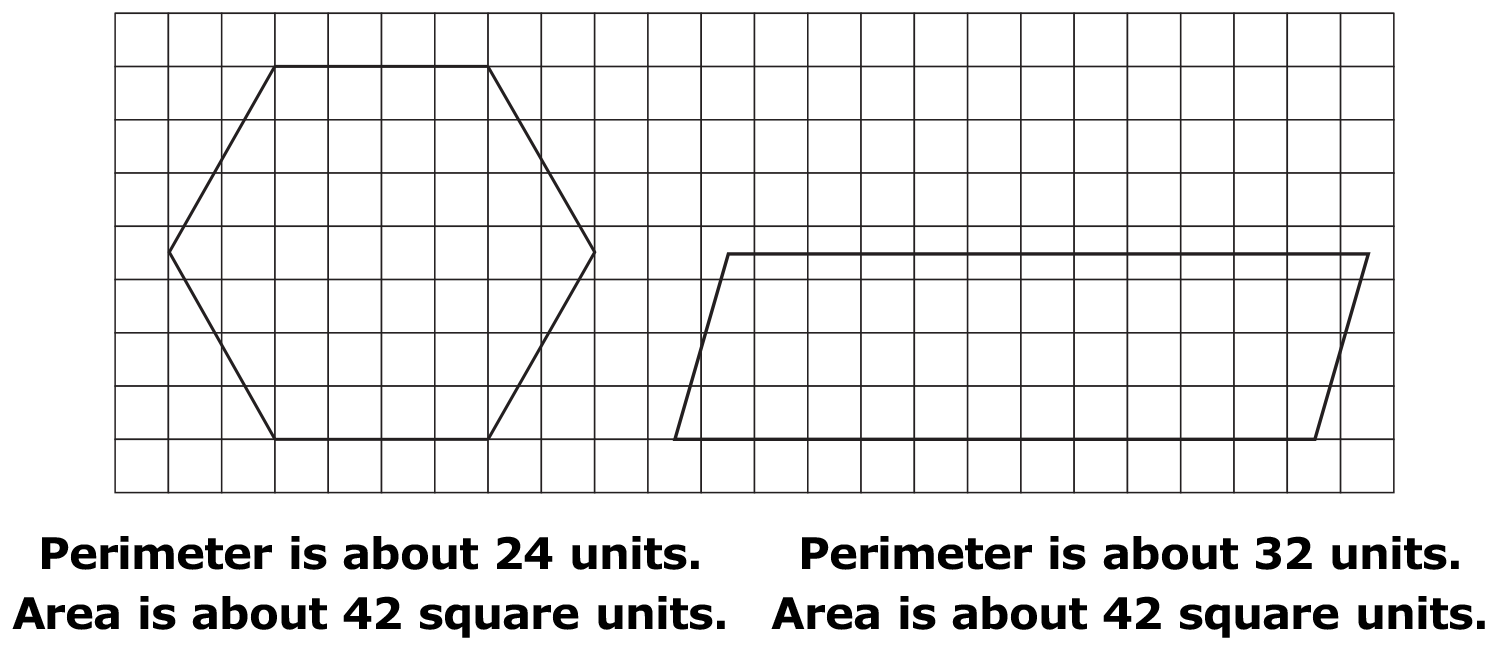From this example, students should recognize that figures with the same area can have different perimeters. Challenge them to draw other figures with an area of 42 square units that have different perimeters.

References
Burns, M. About Teaching Mathematics, Sausalito, CA Van Hiele, P.M. (1984) A Child’s Thought and Geometry

## Computer Graphing Tool

### Many recent studies suggest the use of technology may facilitate students' data organization and graphing skills. The instruction model presented here is an example of using a computer graphing program to achieve this goal.

Step 1: Take a survey.

Step 2: Collect and organize data.
Students collect survey responses and input data into a table.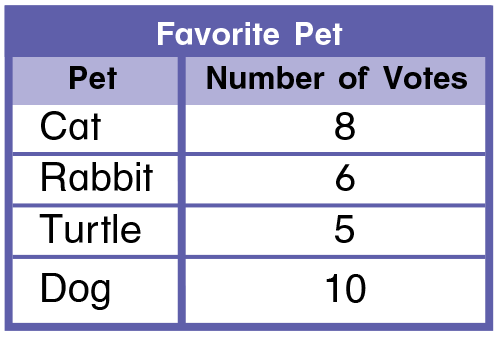Step 3: Create a graph.
Students create a picture graph on the computer by dragging one of the four symbols on the top of the screen (hat, soda, hot dog, and popcorn) into the column with the same label. They continue to add symbols to complete the graph.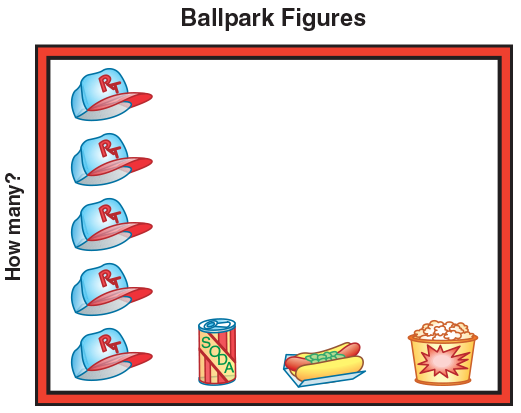Step 4: Choose an appropriate graph.
Students make a bar graph to view the data in a different way. The computer allows for simultaneously viewing of two different graphs. In this situation, students see a bar graph is more efficient in presenting a larger quantity of data.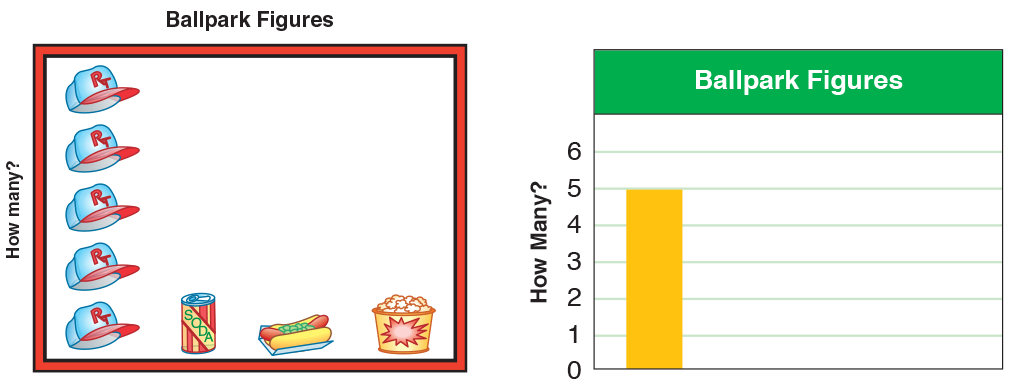There are more options for manipulating and changing the graph on a computer. For example, students may change the scale of the graph by selecting "Scale Maximum" from the graph menu. They may also view other types of graphs by clicking on the small icons at the bottom of the screen.

References
National Research Council, (2001). Adding It Up: Helping Children Learn Mathematics, 255–312
Jones & Thornton, G.A., et al (2000) A framework for characterizing student’s statistical thinking. Mathematical Thinking and Learning, 2, 269–307

## Cup, Counters, and Double Pan Balance

### Much research suggests that students best develop their early algebraic thinking through concrete activities and real-world problem situations. The following instruction model demonstrates a hands-on activity to help develop students’ understanding of variables, expressions, and balanced number sentences.

A veterinarian weighs a cat by putting it in a box on a scale. The box weighs 2 pounds. Students can use a cup and counters to represent the weight of a cat in the box. The cup represents the weight of the cat, which is unknown, and the 2 counters stand for the 2 pounds in the weight of the box.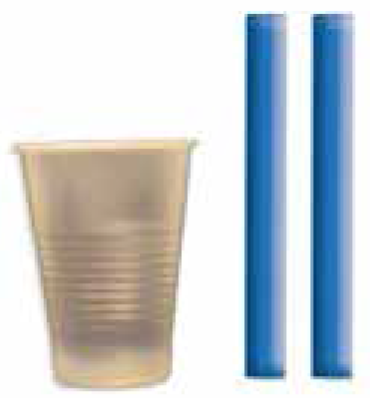To show this general situation symbolically, students can write the expression c + 2, using the variable c to stand for the unknown weight of the cat.

The cup can then be used to demonstrate that a variable can have different values. Place different numbers of counters in the cup to represent different weights of cats.

Students who understand how to represent the general situation are ready for a specific situation, such as when the scale reads 10 pounds. Place an empty cup and 2 counters on one side of a double pan balance and 10 counters on the other side.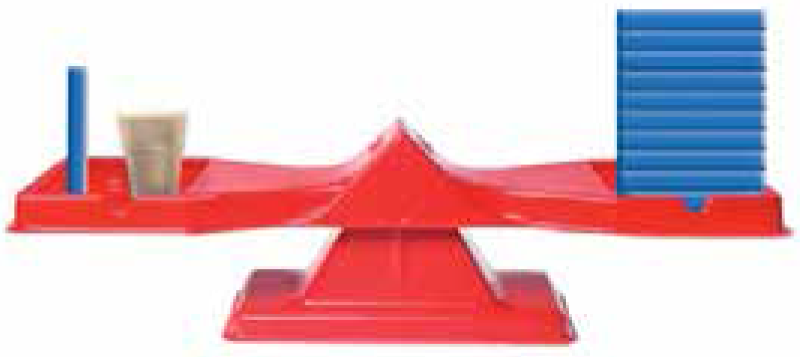To show this situation symbolically, students can write the number sentence 10 = c + 2. For the scale to balance, 8 counters must be put in the cup. So, the value of c in the number sentence must be 8.

Students who understand the concept of a variable and a balanced number sentence will be ready for solving equations.

References
National Research Council, (2001). Adding It Up: Helping Children Learn Mathematics, 255–312
Carpenter, T. P.; Franke, M. L.; Levi, L. (2003) Thinking Mathematically: Integrating Arithmetic and Algebra in Elementary School.
Wickett, M., Kharas, K. & Burns, M. (2002) Lessons for Algebraic Thinking, Grades 3–5.

## Fraction Number Line

### A number line is an effective tool to help students visualize relationships between and among numbers. A fraction number line helps students see fractions as numbers and understand their relationship to 1. Parallel fraction number lines help students see relationships among fractions, including equivalency.

The distance between 0 and 1 on a number line can be divided into fractional parts. The points on that number line can then be named with fractions. The number line below is divided into fourths. It shows how the fractions 14 , 24 , and 34 relate to each other and to 0 and 1. Students can use a single number line to compare fractions with like denominators. Because 14 is to the left of 34 , this number line shows that 14 is less than 34.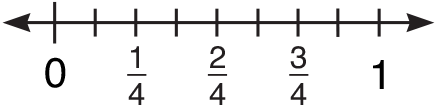Parallel number lines show that the distance between 0 and 1 can be divided into many different fractional parts. The number lines below are divided into halves and sixths. Stu- dents can use a set of parallel number lines to identify equivalent fractions. Because the distance from 0 to 12 is the same as the distance from 0 to 36 , the number lines show that 12 is equivalent to 36 . The fractions 12 and 36 are names for the same point on the number line.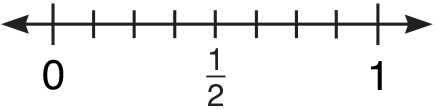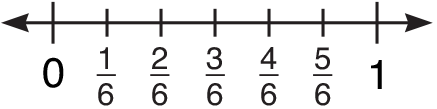Parallel number lines can also be used to compare fractions with unlike denominators. This is especially helpful for those students who have difficulty separating the rules for whole numbers from the rules for fractions. These students may think that 23 is less than 36 because 2 is less than 3 and 3 is less than 6. However, the number lines show that the distance from 0 to 23 is greater than the distance from 0 to 36 . So 23 is greater than 36 .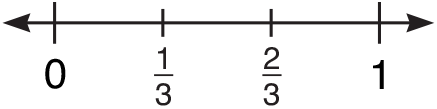Students who have a firm grasp of the meaning of a fraction as part of a whole and the relationship of fractions to each other have the foundation for computing with fractions and working with decimals, percents, and ratios.

References
Burns, M. (2000), About Teaching Mathematics, 223–237, Sausalito, CA
Kamii, C. & Clark, F.B. (1995) Equivalent Fractions: Their Difficulty and Educational Implications. Journal of Mathematical Behavior, v14 n4 p365–78 Dec 1995

## Number Line

Multiplication

### A number line is an effective tool to help students visualize relationships between and among numbers. Young students especially will benefit from “skip counting” on a number line.The following instruction model is aligned with the concrete, pictorial, and abstract pedagogical approach. Here, a number line serves as a pictorial representation of numbers counted. It helps students to bridge the gap from concrete manipulation of objects to the symbolic representation of the numbers.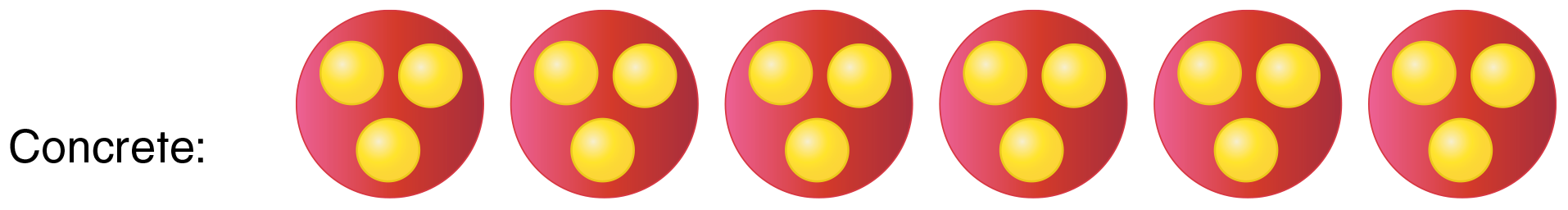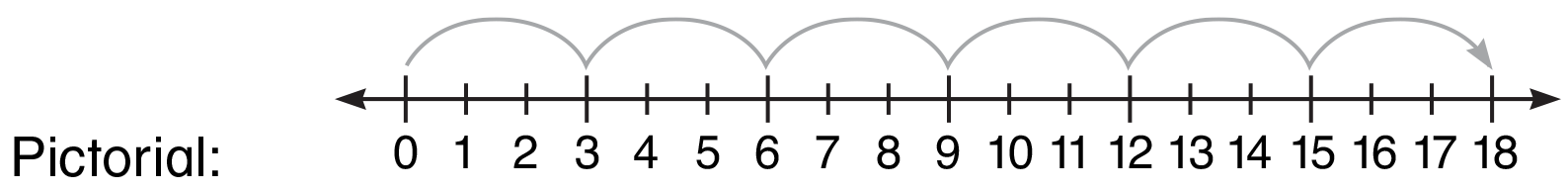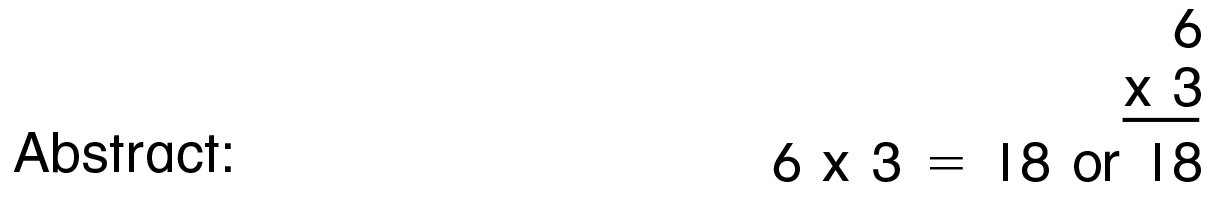Students who understand how to model multiplication on a number line will be able to use the number line to model the order property of multiplication.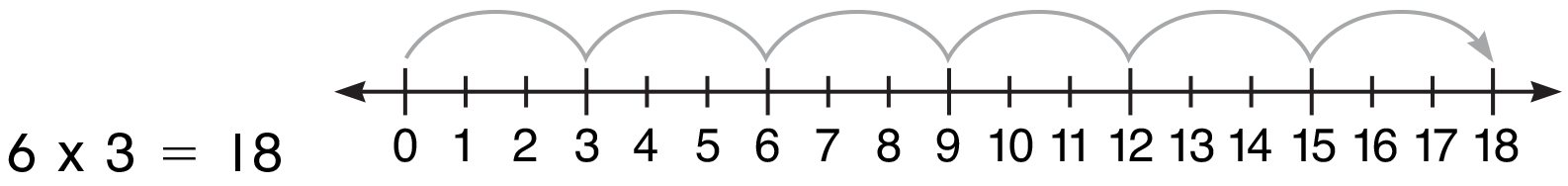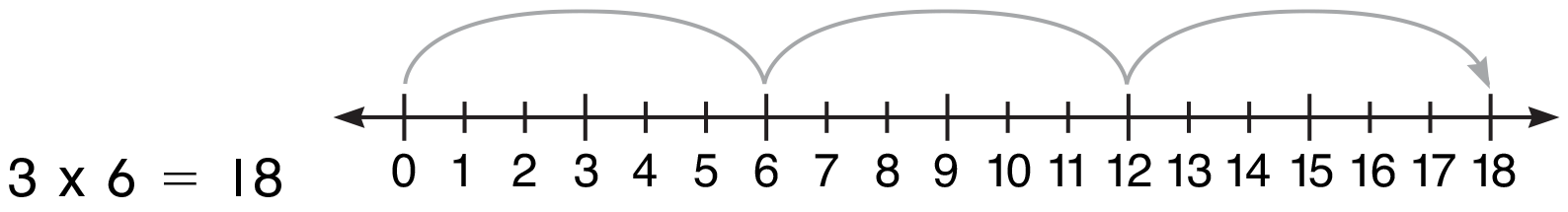They can also use the number line to find missing factors. To find the missing factor in ? × 3 = 18, count the groups of 3 needed to reach 18.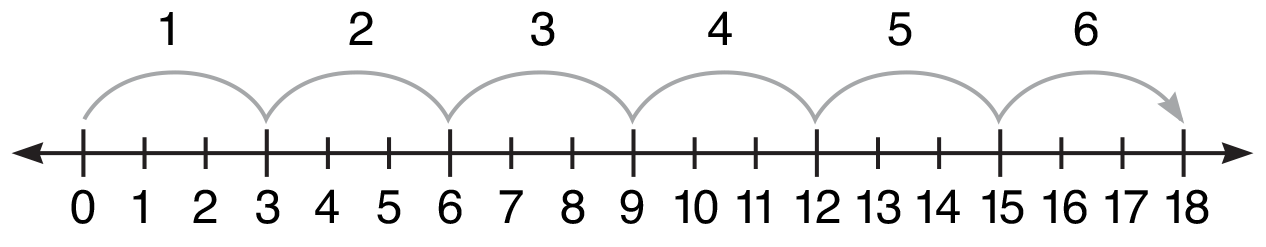References
National Research Council, (2001). Adding It Up: Helping Children Learn Mathematics, 181–229
Baroody, A.J. (1999) The role of estimation and the commutativity principle in the development of third-graders’ mental multiplication. Journal of Experimental Psychology, 74, 157–193
Mulligan, J., & Mitchelmore, M. (1997) Young children’s intuitive models of multiplication and division. Journal for Research in Mathematics Education, 28, 309–330

## Number Line

Division

### Just as a number line is an effective tool for helping students visualize multiplication as repeated addition, it is also an effective tool to help them visualize the inverse operation, division, as repeated subtraction. Division as both sharing and as separating can be modeled on the number line. The following instruction model is aligned with concrete, pictorial, and abstract pedagogical approach. Here students record the work they do with concrete materials on a number line, and then represent it symbolically with numbers.

Example A: Share 12 counters equally by putting an equal number of counters in each of 3 groups. How many counters are in each group?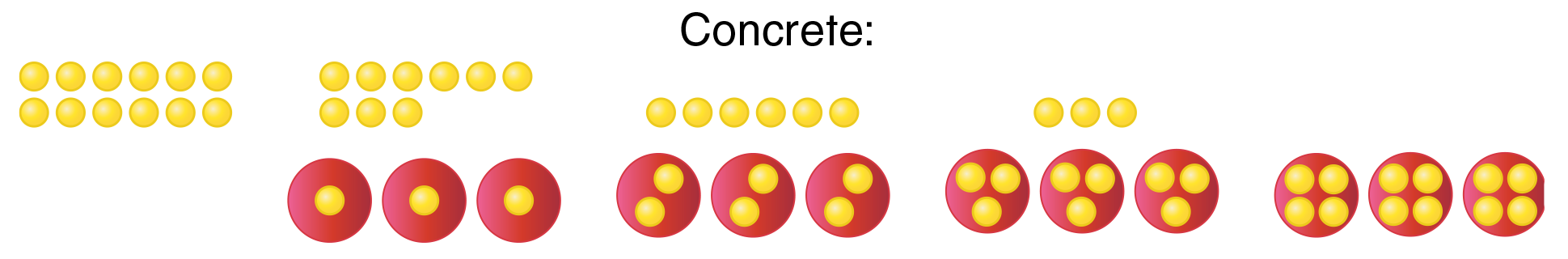As students repeatedly take 3 counters away from the 12 counters to place 1 counter in each of 3 groups, they can record the subtractions on the number line.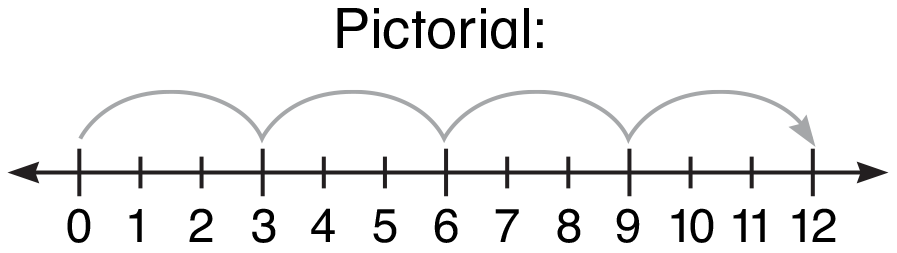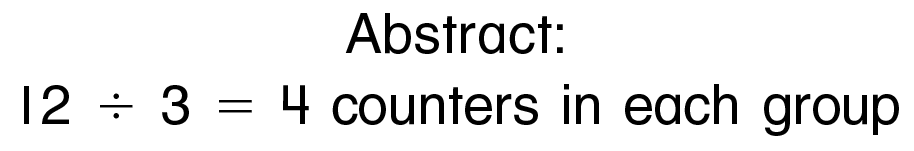Example B: Separate 12 counters into groups of 4. How many groups?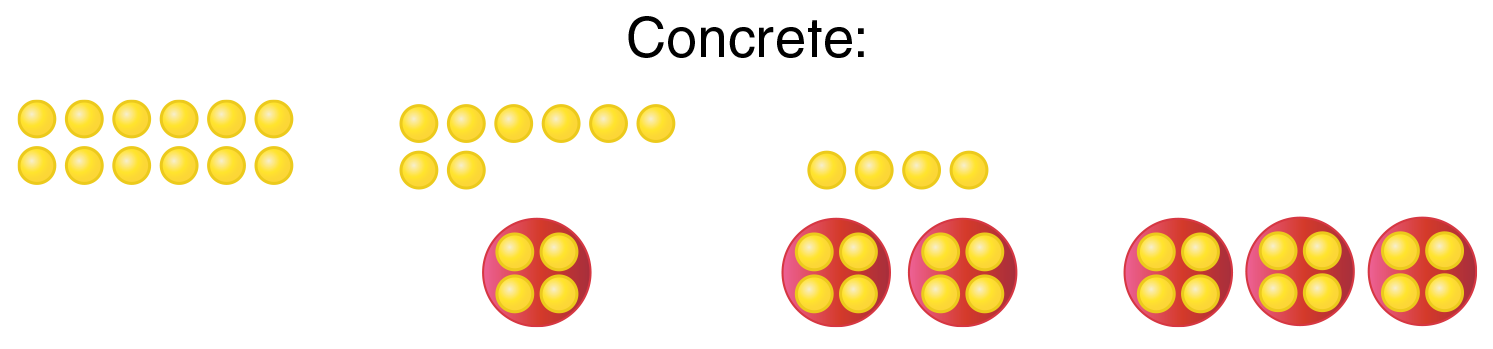References
National Research Council, (2001). Adding It Up: Helping Children Learn Mathematics, 181–229
Thornton, C. A. (1989) Basic Number Facts—Strategies for Teaching and Learning. Book II: Multiplication and Division. An Activity Based Program

## Area Model

### An area model drawn on grid paper is closely related to an array and is also a powerful representation of the multiplication algorithm.

Example A: Use partial products.
The grid below shows the area of a 12 by 8 rectangle. It also models how the product of 12 × 8 is the sum of two partial products by demonstrating the application of place-value concepts, basic multiplication facts, and the Distributive Property of Multiplication over Addition.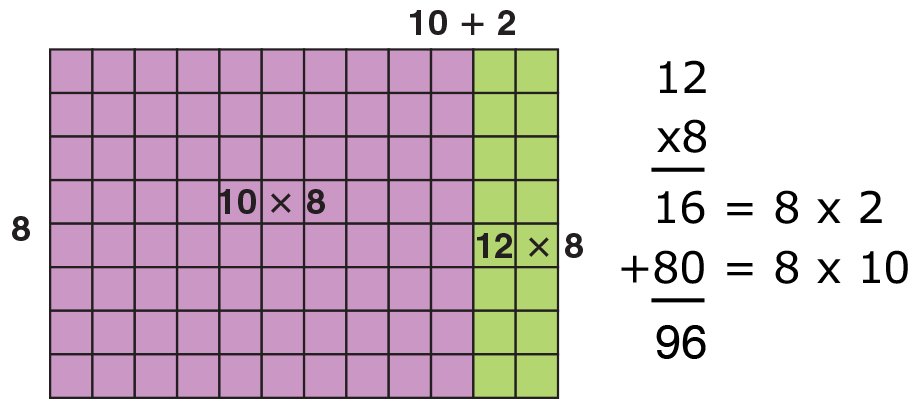This leads to the use of the expanded multiplication algorithm.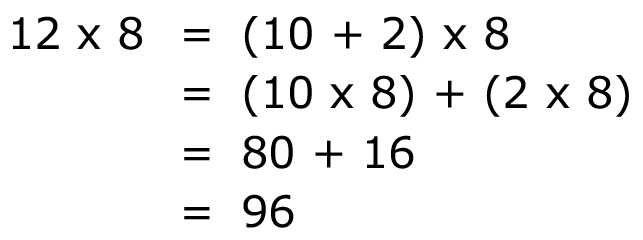Students who understand what each partial product represents in this expanded algo- rithm will be able to easily understand that the traditional multiplication algorithm is simply a shortcut.

Example B: Decompose a factor.
An area model can also be used to represent a different approach to solving 12 × 8. Here the first factor is broken apart to make two basic facts and the Distributive Property of Multiplication over Addition is applied. This approach is aligned with composing and decomposing numbers.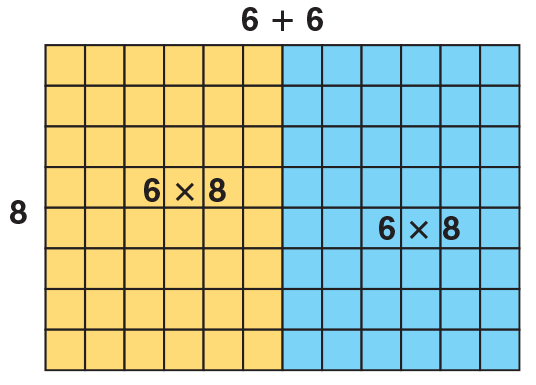References
National Research Council, (2001). Adding It Up: Helping Children Learn Mathematics, 181–229
Fuson, K.C. & Briars, D.J. (1990). Using a base-ten blocks learning/teaching approach for first- and second-grade place value and multiple digit addition and subtraction. Journal of Research in Mathematics Education, 21, 180–206
Carpenter, T.P., Fennema, E., & Franke, M.L. (1996). Cognitive Guided Instruction: A knowledge base for reform in primary mathematics instruction. Elementary School Journal, 97, 3–20

## Array

### An array is a rectangular arrangement of objects with the same number in each row. Various research findings suggest that arrays are powerful representations of multiplication. In this chapter, arrays are used to facilitate students’ thinking strategies for single-digit multiplication and division.

The array below is an example of how this tool can be used to find a product using known multiplication facts. The product of a new combination, such as 7 × 8, can be found by adding the products of the known facts 5 × 8 = 40 and 2 × 8 = 16.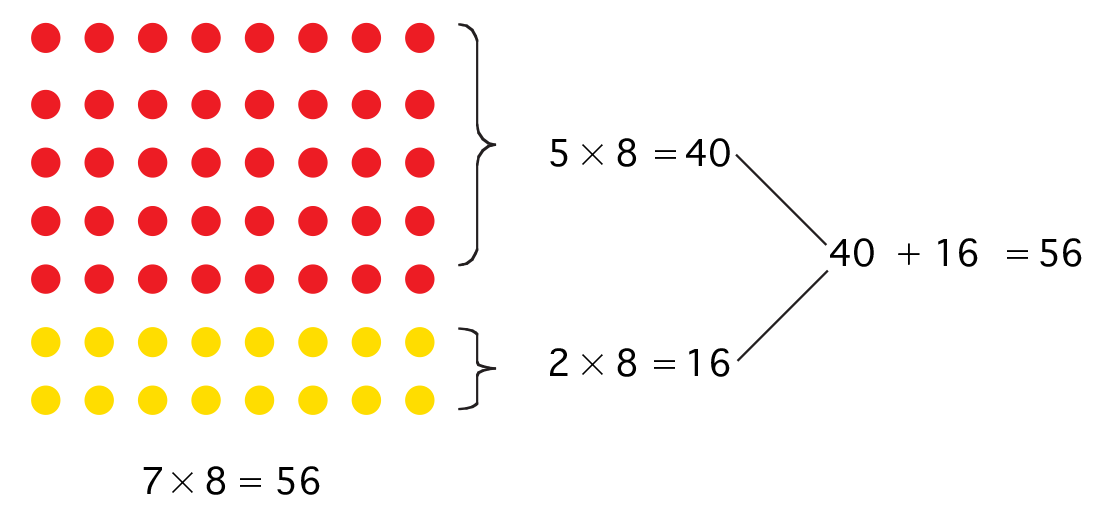Students who understand breaking apart arrays to multiply will be better able to use the basic facts they already know to acquire new basic facts. They will also be prepared to understand the use of partial products to multiply greater numbers.

An array can also be used to facilitate students’ understanding of multiplication and division fact families. For a set of numbers such as 2, 4, and 8, you can write four related multiplication and division facts. Make an array with 2 rows of 4, and write a multiplication fact and the related division fact for the array. Then, turn the array and write another pair of multiplication and division facts. Finally, show students the result of putting the two arrays together to show the equations 4 × 4 = 16 and 16 ÷ 4 = 4.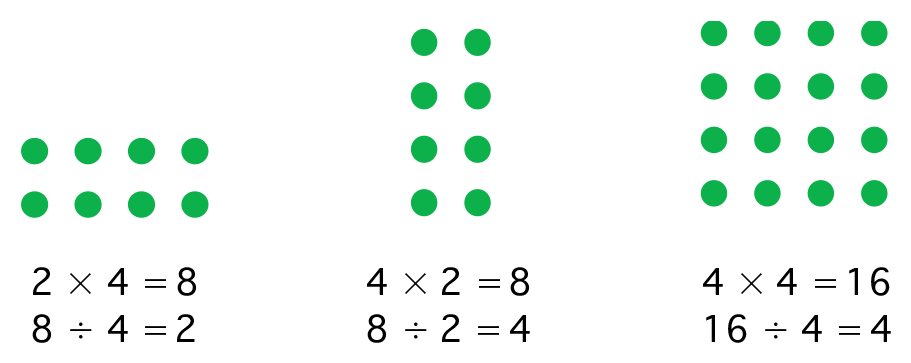Students who understand how to use arrays to model fact families will be able to easily understand why some sets of numbers, such as 4, 4, and 16, have only one representative array. They will also be prepared to eventually understand the concepts of squares and square roots.

## Base-Ten Blocks

Place Value

### Various research findings suggest that sustained experiences with concrete models are needed for students to connect discrete quantities with symbolic representations1. Some researchers further suggest that writing a multi-digit number in various forms facilitates students’ understanding of place value.

The pictorial representation below shows one way to model 138 with base-ten blocks.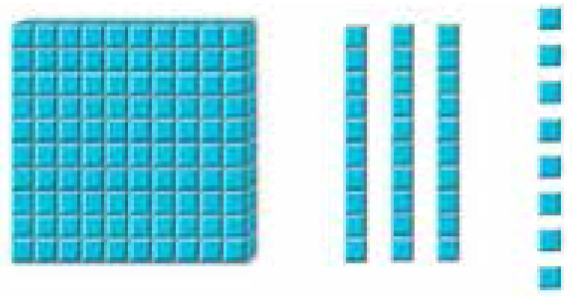By trading a hundred for tens and/or tens for ones, 138 can be modeled in 18 other ways, only two of which are shown below.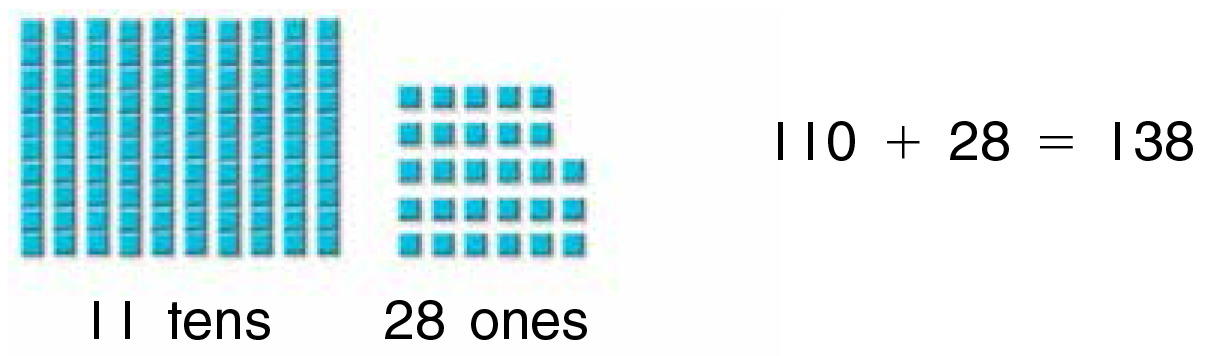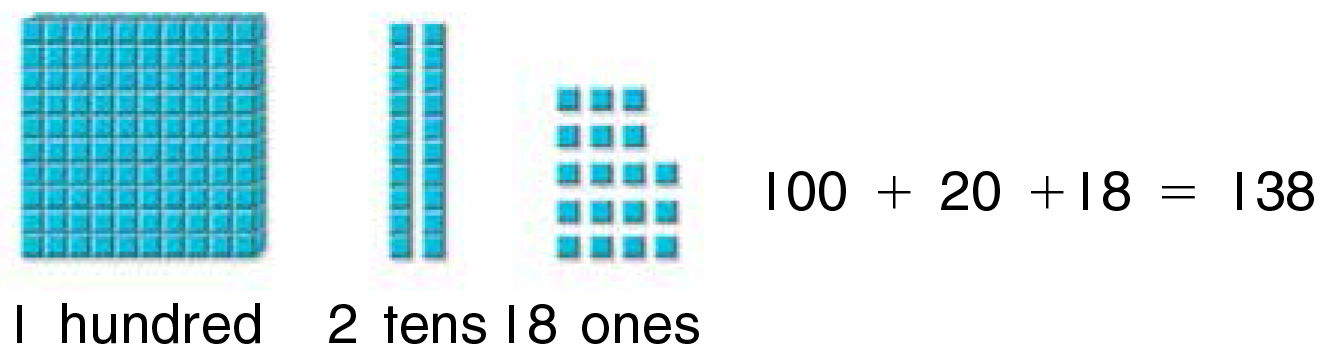To model a number, children should identify the digit in the tens place and lay out that many ten rods. They identify the digit in the ones place and lay out that many ones cubes.

To identify a number represented by a model, children count the number of ten rods to find the digit in the tens place. The number of ones cubes shows the digit in the ones place. Children can also count the total number of ones cubes in the entire model, but this laborious method is time-consuming and ignores the merits of place-value concepts.

References
Van de Walle, J. A. (1998). Elementary and Middle School Mathematics: Teaching Developmentally, 55, 401 New York, NY

## Base-Ten Blocks

Number Sense

### The National Council for the Teachers of Mathematics suggest that students need to have many experiences decomposing and composing numbers in order to solve problems flexibly. For example, 48 can be thought of as 40 + 8, 30 + 18, 50 − 2, 6 × 8, and 4 dozen. Each form may be useful in some particular situation. The following examples demonstrate various strategies of adding multi-digit numbers by decomposing and composing numbers. In each case, students should use base-ten blocks to help them conceptualize the process.

Example A: Break the second addend into parts.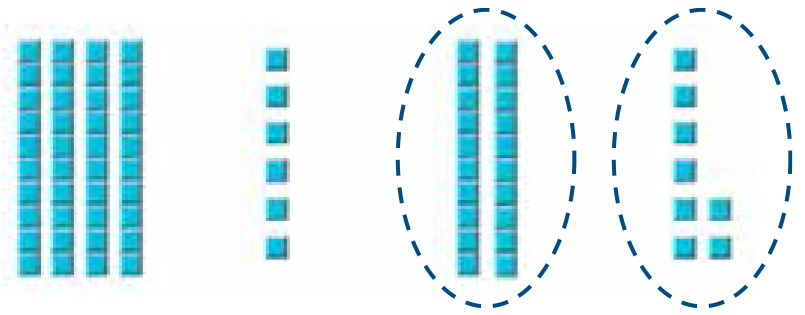Example B: Break apart both addends to add tens and ones separately.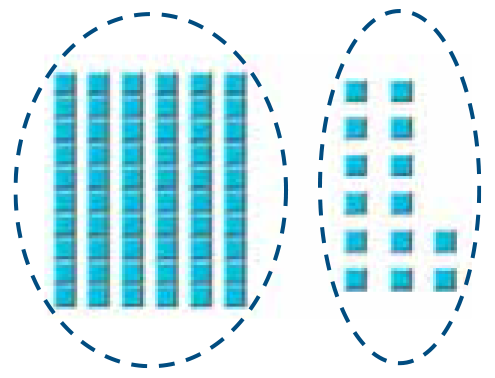Example C: First add numbers to make a ten.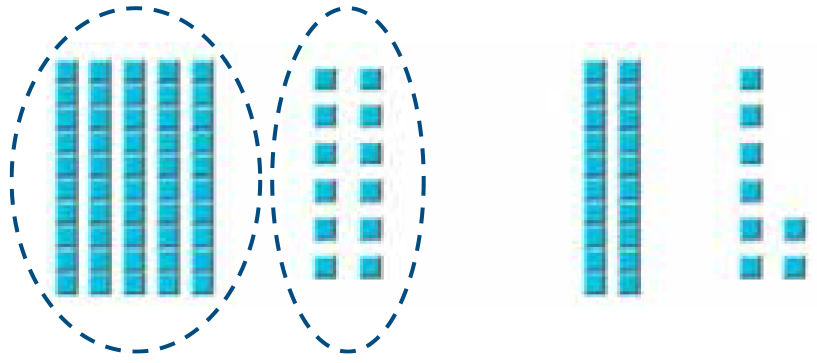Decomposing and composing numbers in different ways will help students understand that the addition algorithm is a shortcut for breaking numbers apart using place value, finding partial sums, and applying mental math strategies.

References
National Research Council, (2001). Adding It Up: Helping Children Learn Mathematics, 181–229
National Council of Teachers of Mathematics (2005), Principles and Standards for School Mathematics
Fuson, K. C. (1992). Korean children’s understanding of multi-digit addition and subtraction. Child Development, 63, 491–506 Singapore Math Series, Singapore, Department of Education

## Base-Ten Blocks

Division Algorithm

### The following instruction model shows how the division algorithm can be taught through concrete models. Here, students record the work they do with base-ten blocks, representing it symbolically with numbers.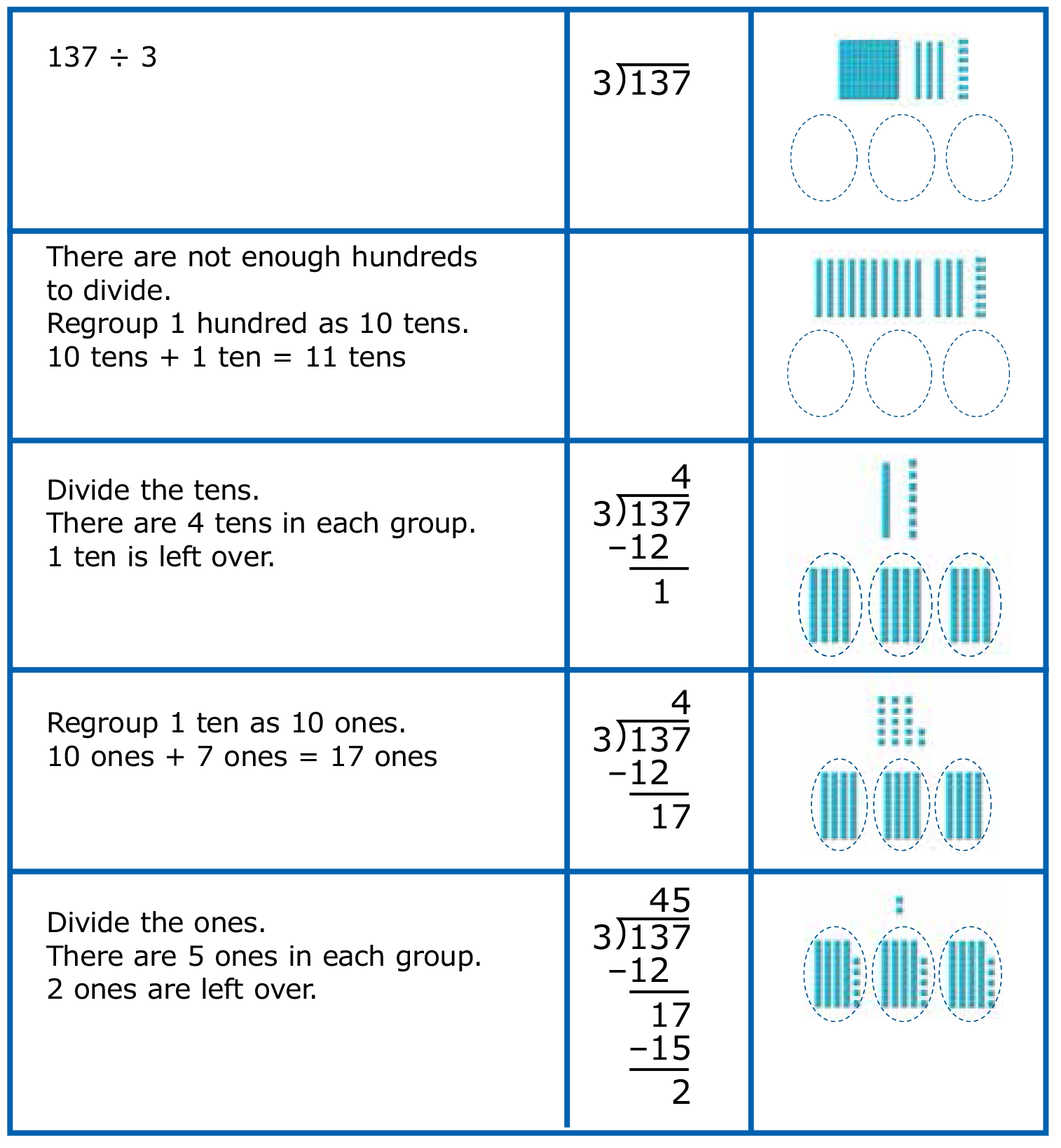Students who grasp this relationship of concrete to abstract will have the foundation to apply the division algorithm to greater whole numbers as well as to rational numbers.

References
National Research Council, (2001). Adding It Up: Helping Children Learn Mathematics, 181–229
McClain, K., Cobb, P., & Bowers, J. (1998) The Teaching and Learning of Algorithm in School Mathematics, NCTM Yearbook
Lampert, M. (1992) Teaching and learning long division for understanding in school. The analysis of arithmetic for Mathematics Teaching, 221¬–282, Hillsdale, NJ

## Bundles of Sticks

### Students need many experiences decomposing and composing numbers in order to solve problems flexibly. The following example demonstrates a strategy of breaking numbers apart to subtract.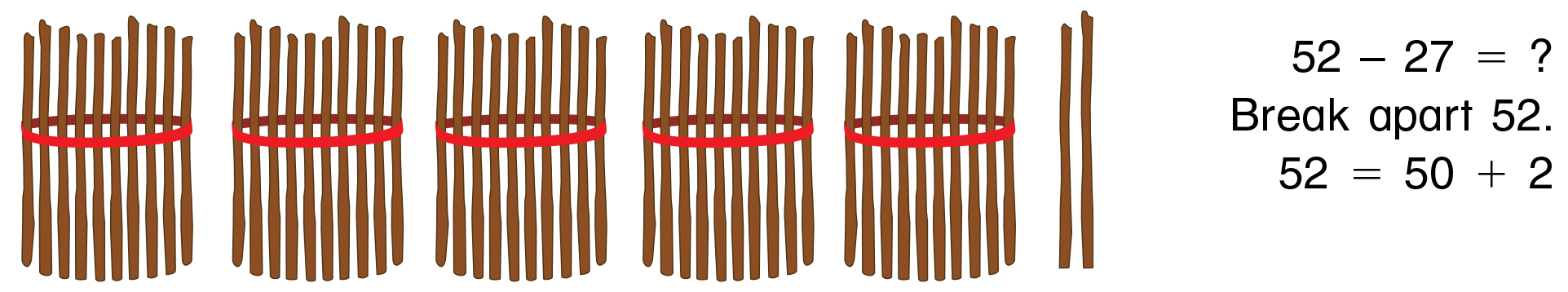Get ready to subtract. You have enough bundles to subtract 20, but you don’t have enough loose sticks to subtract 7. So, break apart a bundle of sticks.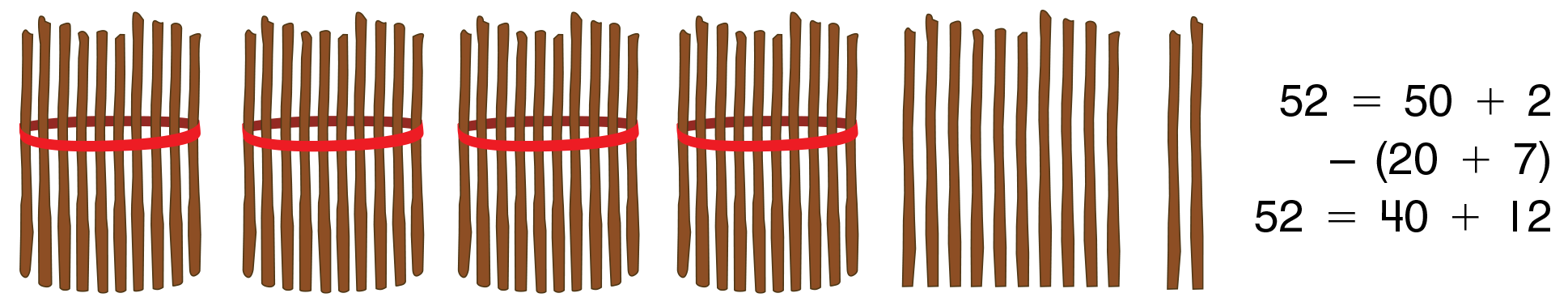Now, subtract 20 + 7.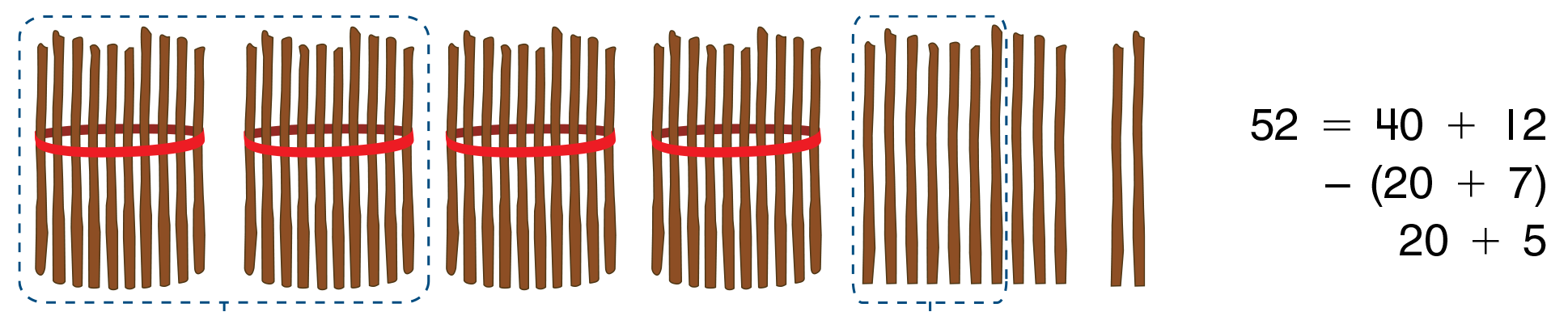25 are left.

Students who understand how to decompose numbers before subtracting them will be able to easily understand why the subtraction algorithm is a shortcut for breaking numbers apart using place value, and why regrouping is needed in certain cases before subtracting. This may also simplify the case of subtracting with zero(s).

References
National Research Council, (2001). Adding It Up: Helping Children Learn Mathematics, 181–229
National Council of Teachers of Mathematics (2005), Principles and Standards for School Mathematics
Fuson, K. C. (1992). Korean children’s understanding of multi-digit addition and subtraction. Child Development, 63, 491–506 Singapore Math Series, Singapore, Department of Education

## Money and Decimal Squares

### Some students benefit from being able to visualize decimals. Pennies and dimes are effective concrete models for their fractional relationships to dollars, and students have already used place-value charts to show money amounts. Decimal squares are effective pictorial models for helping students see the relationships between decimals and common fractions. Used together with place-value charts, money and decimal squares help students bridge the gap from concrete models to the symbolic representation of decimal and fraction equivalency.

If a decimal square is used to represent 1 dollar, then each column represents 1 dime, and each small square represents 1 penny.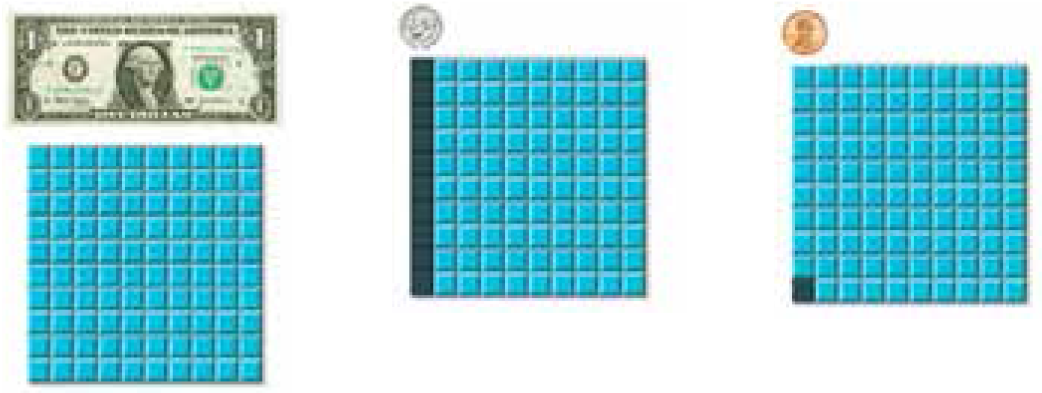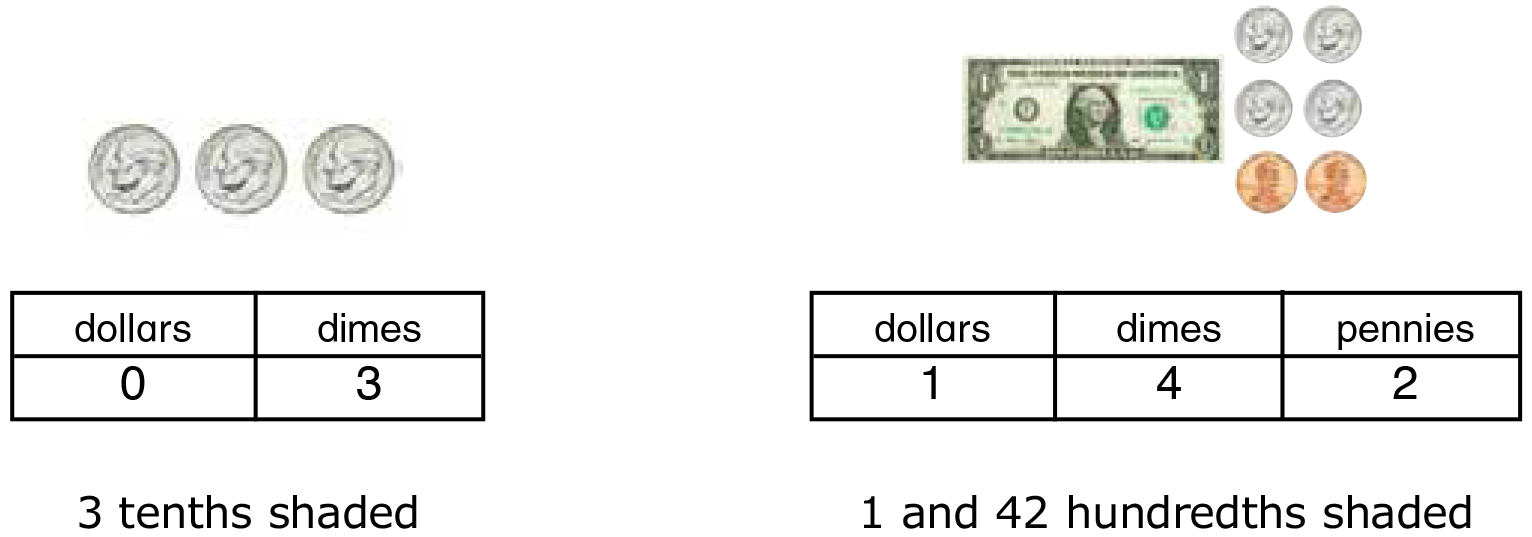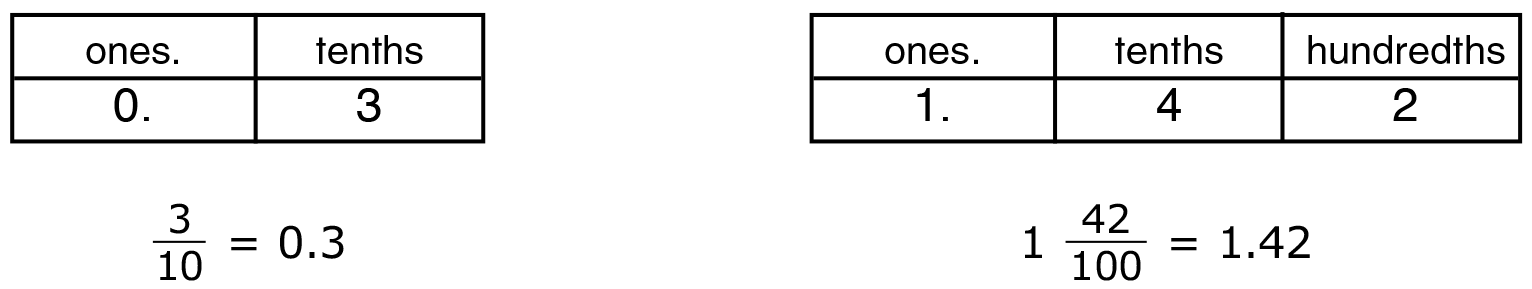References
Burns, M. (2000), About Teaching Mathematics, 223–237, Sausalito, CA
Pagni, D. (2004), Fractions and Decimals, Australian Mathematics Teacher, v60 n4 28-30, 2004

## Standard and Nonstandard Measuring Tools

### Some research findings suggest that it is necessary for young children to recognize the need to use identical units in measurement and understand that each measurement unit could be partitioned into smaller parts. Research also suggests that many children respond to linear measurement with a nonzero origin by simply reading off whatever number on a ruler aligns with the object.

Provide students with a set of three lines drawn on paper such as, 3 58 inches, 6 132 inches, and 5 38 inches long; three types of pasta, such as curly, elbow, and ziti; and an inch and centimeter ruler.

Have students line up pieces of pasta to measure each line and record their measure- ments in a table. Discuss why different types of pasta are nonstandard units of measure.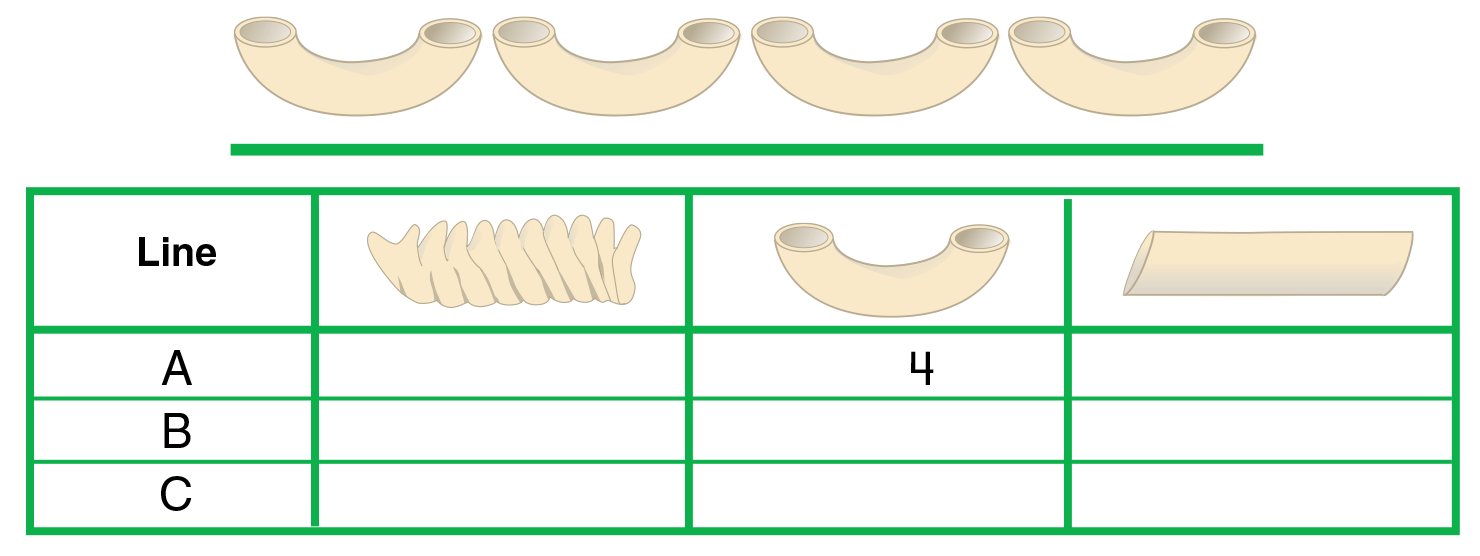The same lines can be used to measure to the nearest inch, half inch, and quarter inch. Discuss why an inch is a standard unit of measure. Check that students are aligning the zero end of their rulers with the beginning of each line.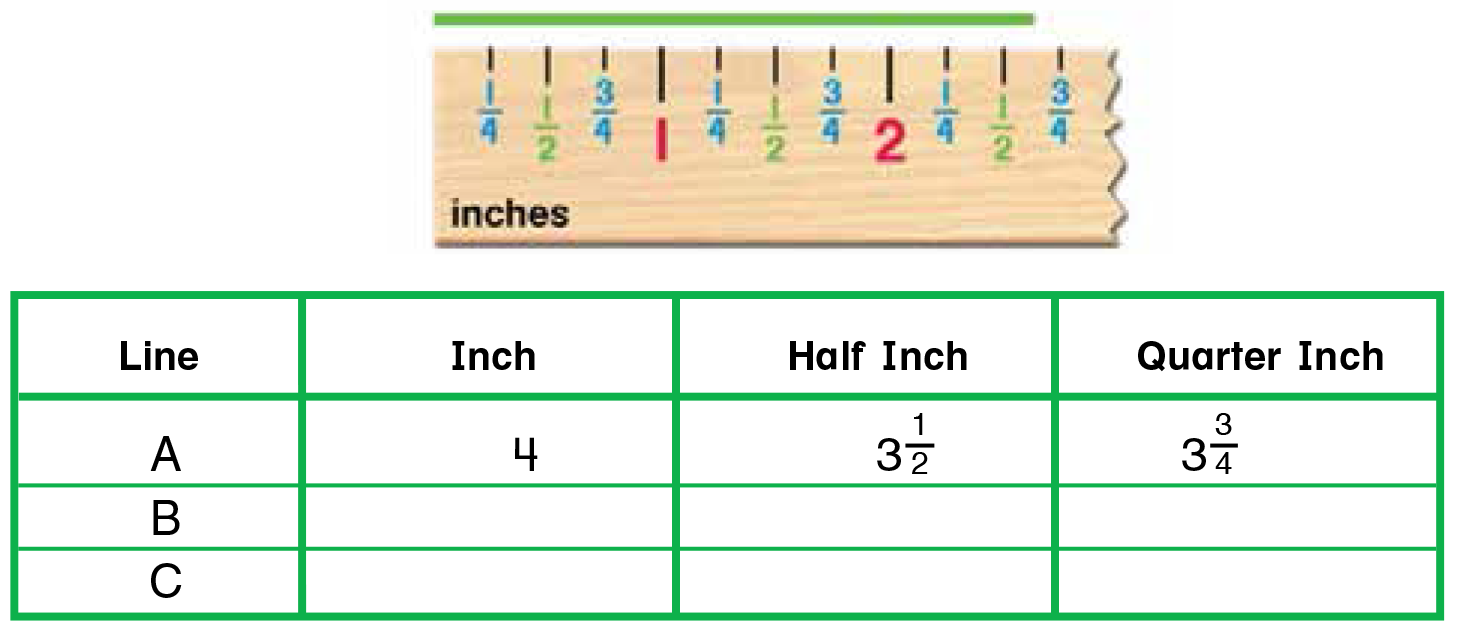Then, have students measure each line by aligning the 1-or 2-inch mark with the beginning of the line. Have them compare these measurements with the first set of measurements. Discuss how their work on this task was different.

References
Lindquist, M. (1998) The measurement standards. The Arithmetic Teacher, 37, 22–26
Lehrer, R., Jenkins, M., & Osana, H. (1998) Longitudinal study of children’s reasoning about space and geometry. Designing learning environments for developing understanding of geometry and space, 137–167, Mahwah, NJ: Erlbaum